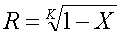Country rankings are based on an aggregation of the TopCoder members within a particular country that have competed within the last 180 days, in other words, those that are "active".

The formula below has the property that if all the coders from a particular country have the same rating, then that country's rating would be the same as the individual ratings. When coder ratings differ, the country rating is based mostly on the top rated coders. The intent is to provide a better way to rank countries than a simple average.

When generating the country rankings we take the individual ratings for active coders from a particular country, sorted in decreasing order. These ratings are given by rating1 ... ratingM, where M is the total number of active coders from a country.Where:
M is the number of active coders from a particular country
i is a 1 based index of active coders from a particular country when sorted by rating in descending order, in other words, their rank within their country
ratingi is the rating of the coder with index i
R is a constant that will dictate the weighting of the different ranks

One may determine the weighting (R) using the following equation.Where X is the fraction of a country's rating that will be based on the top K coders within that country.

We have chosen an R of .87, this means that the top 10 coders contribute about 75% of a country's rating.

Rating formula courtesy of ChristopherH